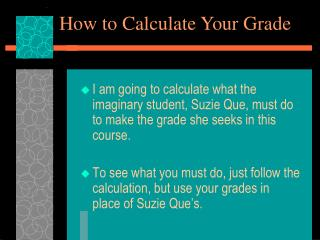DownloadDownload PresentationI am going to calculate what the imaginary student, Suzie Que, must do to make the grade she seeks in this course.

# I am going to calculate what the imaginary student, Suzie Que, must do to make the grade she seeks in this course.

Télécharger la présentation## I am going to calculate what the imaginary student, Suzie Que, must do to make the grade she seeks in this course.

- - - - - - - - - - - - - - - - - - - - - - - - - - - E N D - - - - - - - - - - - - - - - - - - - - - - - - - - -
##### Presentation Transcript

1. How to Calculate Your Grade • I am going to calculate what the imaginary student, Suzie Que, must do to make the grade she seeks in this course. • To see what you must do, just follow the calculation, but use your grades in place of Suzie Que’s.

2. First I am going to determine how many bonus points Suzie will get. Remember there are more than enough pop quizzes to get a maximum of 40 bonus points. I am going to assume that Suzie’s pop quizzes gets her 35 points.

3. Next I need to estimate what Suzie’s lab experiment average will be at the end of the semester. • I estimate that to be 97. • So far she has 97+35=132 points.

4. Now I must add in her two lecture exams so far. • For example, let’s assume Suzie got 31 correct on the first test and 36 correct on the second. (Each test had 50 questions.) • Let’s see how many points that gets her. • Her total is now 93+108+132=333.

5. Let’s assume that she wants to make a “B.” That requires 640 (actually 630) total points. • 630-333=297 • 297 points on the remaining exams is required to make a “B.”

6. There is still 400 points available on the remaining exams (this includes the lab final). • In this example Suzie can make a middle “C” (74.3%) average on the next two exams and lab final and get a “B” in the course.

7. Please note that in this example Suzie’s best test score on the first two lecture exams was 36. • This gives her a % grade of • Thus you can see that Suzie still has a chance for a “B” in the course even though she made a low “D” and a low “C,” respectively, on the first two lecture exams.